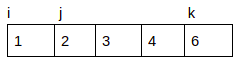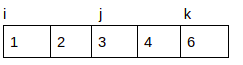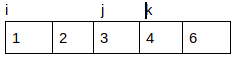×

Search anything:

# 3 Sum Smaller Problem

#### Algorithms Interview Problems on ArrayGet this book -> Problems on Array: For Interviews and Competitive Programming

The 3 sum smaller problem is an interesting algorithm problem that we shall be solving in this article. This is an extension of the 3 Sum Problem. In this, we have to select 3 different elements from an array such that the sum is less than a target.

## Table of Content

1. Examining the Problem Statement
2. Solving the problem
1. The Straight-Forward Approach
2. The Two-Pointer Technique
3. Conclusion

Prerequisites: Two Pointer Approach, 3 Sum problem

## Examining the Problem Statement

Before we dive into the solution, let us take a second to properly define the problem statement and ensure that we have a good understanding of the problem.

Given an array of n integers numbers and a value, find the number of index triplets i, j, k with 0 <= i < j < k < n such that array[i] + array[j] + array[k] < target.

So our algorithm takes in an array of numbers and defined values and we then proceed to calculate the number of index triplets (index triplets meaning even if a number is repeated we should still count it if it is a part of a valid triplet) and return it.

For example, if we are given an array, [2,3,7,5] with a value 14. The number of triplets that can be gotten from the array that their sum will be less than 14 is 2 i.e (2,3,5) and (2,3,7). Now that we have a clearer understanding of what the problem entails we can proceed to solve it.

## Solving the Problem

There are two approaches to solving the 3-sum smaller problem. The straight-forward approach and the two-pointer technique.

### The Straight-Forward Approach

This is a brute force technique. Here we shall use 3 for-loops that we will use to try every possible combination of triplets and count the ones that fulfil our criteria, i.e that sum up to less of the given value.

For example, if we had an array [2,3,7,5] and we wanted to get all possible triplets starting from 2 we would get (2,3,7), (2,3,5), (2,7,5), (3, 7, 5)

### Implementation

``````def sumSmaller(lst, num):
"""
returns the number of triplets contained in the array whose sum
is less than num
"""
count = 0 # initialise the counter

# starting from the first index get all the possible combination of triplets
for i in range(len(lst)):
for j in range(i + 1, len(lst)):
for k in range(j + 1, len(lst)):
if sum((lst[i],lst[j],lst[k])) < num:
count += 1
return count
``````

### Time Complexity Analysis

Although the straight forward approach is intuitive, it is not the best algorithm we can use to solve the problem. It has a time complexity O(n^3) because of the 3 for-loops that each span through the whole array, each averaging n amount of work. We can do better as will be soon be seen.

### The Two-Pointer Technique

This technique requires that we sort the array first (you will see why in a second). We first initialise a counter. Then using a single for loop we iterate through the array starting from the first element and on each iteration, we maintain 2 pointers j and k. such that j is equal to the current loop index i plus 1, i.e j = i + 1 while k is set to the end of the array, i.e k = length(arr) - 1.

Then while k is greater than j if array[i] + array[j] + array[k] is less than the given value increment j by 1 and add all the total number of indices between j and k(j inclusive) to the count but if array[i] + array[j] + array[k] is greater that the given value then decrement k by 1.

Let's illustrate with an example to make the explanation clearer. Given an array [2, 3, 1, 4, 6] and a value of 10. First, we sort the array.
Then iterating from i = 0, we set j to 1 and k to 4 and at first count is 0since array[i] + array[j] + array[k] is less than 10 we add 3 (k - j) to count. We then increment jarray[i] + array[j] + array[k] is greater than 10 so we decrement karray[i] + array[j] + array[k] is less than 10 we add 1 to count and then increment j. K is no longer greater than j and hence the inner loop is terminated and we start another ith iteration. The algorithm eventually terminates after the last iteration.

### Implementation

``````def sumSmaller(lst, num):
"""
returns the number of triplets contained in the array whose sum
is less than num
"""
lst.sort()
count = 0 # initialise counter

# starting from the first index add up the number of valid triples
for i in range(len(lst)):
j = i + 1
k = len(lst) - 1
while j < k:
if sum((lst[i], lst[j], lst[k])) < num:
count += k - j
j += 1
else:
k -= 1
return count
``````

### Time Complexity Analysis

We first perform a sort operation that has a time complexity of O(nlogn). In the for-loop, we iterate from i = 0 to n and in each iteration the while loop is averaging n amount of work, giving us a time complexity of O(n^2). The total time complexity of the function is given as O(n^2). The two-pointer technique is superior to the straight forward approach and is the best we can get for the problem.

## Conclusion

We have been able to look at two approaches to solving the 3 sum-smaller problem. The straight-forward approach and the two-pointer method. We have also been able to see that sometimes the first or most intuitive approach to solving a problem might not be the most efficient. 3-sum smaller is a modification of the 3 sum problem, if you would like to also see how to approach the 3 sum problem you can refer to this article.

#### Thompson Mina

Student at University of Lagos, Nigeria | Wolves are my spirit animals